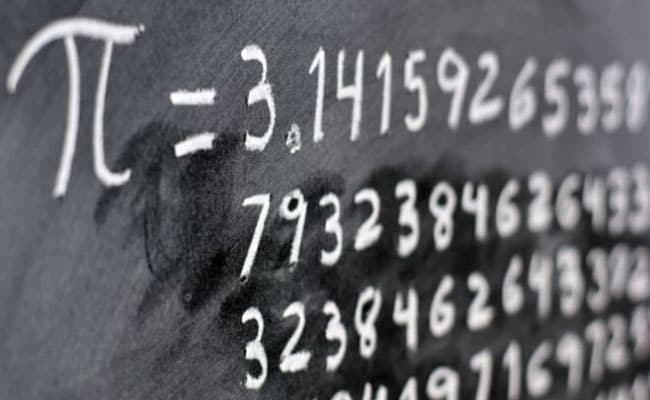# Pi Day: History, Significance And Other Things You Need To Know

0

## Pi Day celebrates the the mathematical constant, Pi. The day is also celebrated as the birthday of Albert Einstein.Pi is actually an irrational number that is most often approximated with the decimal 3.14.

It’s a special day for those who love mathematics and want to honour Albert Einstein. March 14 every year is celebrated as Pi Day to commemorate mathematical constant Pi. It is also the birthday of Einstein, one of the greatest physicists of all time.

Einstein was born on Pi day, March 14, 1879.

What is Pi Day?

Pi Day is held every year on March 14 to celebrate the mathematical constant, Pi (π). The date, when written in the format of month/day (3/14), matches the first three digits of the mathematical constant.

An entire website is dedicated to Pi Day, which also defines the mathematical constant as the ratio of a circle’s circumference to its diametre.

Pi is actually an irrational number that is most often approximated with the decimal 3.14 or the fraction 22/7.

It has interested people around the world for over 4,000 years. Many mathematicians – including famous ones such as Fibonacci, Newton, Leibniz, and Gauss – have toiled over Pi, calculated its digits, and applied it in numerous areas of mathematics.

Pi is a part of Egyptian mythology. People in Egypt believed that the pyramids of Giza were built on the principles of pi.

History

The value of the Pi was first calculated by Mathematician Archimedes of Syracuse. It was later accepted by the scientific community when Leonhard Euler used the symbol of Pi in 1737.

The first Pi Day was celebrated in 1988 by American physicist Larry Shaw. As part of the celebrations, people also eat pie on this day or participate in competitions.

The celebrations

Pi Day is celebrated by lovers of mathematics every year by Pi recital competitions and Pi Day workouts to stimulate interest in learning and practising mathematics.